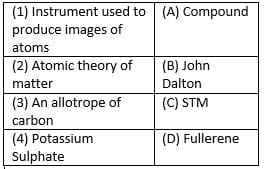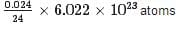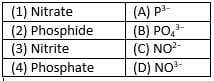# HOTS Questions: Atoms and Molecules

## 25 Questions MCQ Test NCERT Textbooks (Class 6 to Class 12) | HOTS Questions: Atoms and Molecules

Description
Attempt HOTS Questions: Atoms and Molecules | 25 questions in 25 minutes | Mock test for UPSC preparation | Free important questions MCQ to study NCERT Textbooks (Class 6 to Class 12) for UPSC Exam | Download free PDF with solutions
QUESTION: 1

### Who suggested that if we go on dividing matter, a stage will come when particles obtained can’t be divided further?

Solution:

Democritus and Leucippus suggested that if we go dividing matter, a stage will come when particles obtain can’t be divided further.

QUESTION: 2

Solution:

Cobalt - Co
Copper - Cu
Sulphur - S
Sodium - Na
Hence, option B.

QUESTION: 3

### What information we get from molecular formula A It represents one molecule of the substance  B It does not tells the name of the substance  C It tells about the type of atoms  D It represents formula mass unit of one substance

Solution: The chemical formula can tell you the amount of each individual element that makes up that compound. Usually, they are derived by mass, so if you multiply the subscript number by the elemental mass of the number preceding, you can find how much of that element is in that compound.
QUESTION: 4

One mole of N2 is equal to-

Solution:

B is the correct option.In order to have one mole of things, let's say particles, you need to have 6.022⋅1023 particles → this is known as Avogadro's constant and acts as the definition of the mole. So, in one mole of nitrogen gas you have 6.022⋅1023 molecules of nitrogen gas, N2

QUESTION: 5

Atom of Hydrogen is

Solution:

The diameter of Hydrogen atom is 10−10m. it is the smallest atom among all elemetns.

QUESTION: 6

Match the following with correct response.Solution:

STM ( scanning tunneling microscope)  is  an instrument for Imaging surfaces at the atomic level.
John Dalton gave the Atomic theory of Matter.
Fullerene is an Allotrope of carbon.
Potassium sulphate is a compound.

QUESTION: 7

How many moles are present in 40 g of He?

Solution:

Number of moles = mass of the substance molar mass. = 40/4 = 10 moles. Atomic mass or molar mass of Helium is 4 u.

QUESTION: 8

Calculate the number of Mg atoms in 0.024 g of Mg

Solution:

Atomic mass of Mg is 24. So, 24 g of Mg contains 6.022 x 1023 atoms. No. of atoms in 0.024 g of Mg == 6.022 x 1020 atoms.

QUESTION: 9

Who was the first to use the symbols for elements?

Solution:

Dalton was the first scientists who use the symbols for elements. The modern symbols are different from symbol proposed by Dalton.

QUESTION: 10

What according to Dalton’s atomic theory is true among the following-
A. Atom is divisible into Protons, electrons and neutrons
B. Atoms of the same element have different atomic masses.
C. Atoms are the ultimate indivisible particle of matter
D. Atoms of same element have same atomic masses size and chemical properties

Solution:

The correct answer is B as  all the statements of option c and d are true about dalton's theory .

QUESTION: 11

Which of the following elements are present in Quick lime?

Solution:

Chemical formula of Quick lime is CaO. So, elements present in Quick lime is Calcium and Oxygen.

QUESTION: 12

Statement A: mole is quite often known as chemists dozen
Statement B: the mass of one twelfth (1/12) of the mass of one atom of carbon taken as 1u.
Which of the two statement is true

Solution:

Mole is also known as chemist dozen. The mass of one twelfth of the mass of one atom of carbon taken as 1u. So, both the statements are correct.

QUESTION: 13

What is the Latin name of Potassium?

Solution:

Latin name of Potassium element is Natrium from which symbol of Sodium is derived as Na.

QUESTION: 14

During a chemical reaction, the sum of the masses of the reactants and products remains unchanged. This is known as

Solution:

Law of conservation of mass states that, during chemical reaction, the sum of the masses of the reactants and products remains unchanged.

QUESTION: 15

What is the formula of calcium phosphide?

Solution:

Chemical formula of Calcium phosphide is Ca3P2. Valency of Calcium is 2 and Phosphate is 3.

QUESTION: 16

How many molecules are present in one gram molecular mass of a substance?

Solution:

The number of molecules present in one gram molecular mass of a substance is 6.022 × 1023 molecules called as Avogadro number.

QUESTION: 17

How many atoms are present in PO4−3ion?

Solution:

There are 5 atoms in the given ion, in which 1 atom is of Potassium and 4 atoms of Oxygen.

QUESTION: 18

Statement A: Atoms can exist independently
Statement B: The law of constant proportions is applicable only to pure chemical compounds.
Which of the two Statements is true?

Solution:

An atom cannot exist independently. The law of constant proportions is applicable only to pure chemical compounds. So, only statement B is correct.

QUESTION: 19

What is the mass of 0.5 mole of Hydrogen atom

Solution:

Atomic mass of hydrogen is 1. So, one mole of Hydrogen is equal to 1 g. Mass of 0.5 mole of Hydrogen atom will be 0.5×1=0.5g.

QUESTION: 20

The atomic mass of calcium is 40 the number of moles in 60 g of calcium are

Solution:
Number of moles of Ca = Given mass of calcium / Molar mass of calcium = 60 / 40 = 1.5 moles.
QUESTION: 21

What is the name given to the short hand representation of an element?

Solution:

Short hand representation of an element is called symbol. Symbol of an element is derived from English name or Latin name.

QUESTION: 22

Which postulates of Dalton’s atomic theory is the basis of the law of conservation of mass?

Solution:

The Dalton’s postulates that Atoms can neither be created nor destroyed are the basis of law of conservation of masses.

QUESTION: 23

How many moles are present in 2.3 g of Na

Solution:

Molecular mass of Sodium is 23 u. So, 23 g of sodium is equal to 1 mole. The number of mole in 2.3 g = 2.3 / 23 = 0.1 mole.

QUESTION: 24

Match the following with correct response.Solution:

A is the correct option.
Nitrate is a polyatomic ion with the chemical formula NO3- Salts containing this anion are called nitrates.
The chemical formula of Phosphide is P-3.
The nitrite ion, which has the chemical formula NO⁻ ₂. Nitrite is widely used throughout chemical and pharmaceutical industries.
In chemistry, a phosphate is an anion, salt, functional group or ester derived from a phosphoric acid. PO₄³⁻

QUESTION: 25

What is the latin name of sodium?

Solution:

Latin name of sodium is Natrium. Therefore, symbol of Sodium is Na.Use Code STAYHOME200 and get INR 200 additional OFF Use Coupon Code